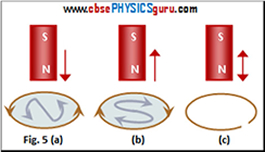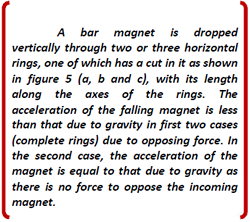Lenz’s Law and Conservation of Energy

According to this law,

The direction of induced emf is such that it tends to produce a current which opposes the change (in magnetic flux) that causes the induction.In figure 1(a), the North-pole of the bar magnet is moved towards the coil. This increases the magnetic flux through the coil. According to Faraday's law, current is induced in the coil. But according to Lenz's law, induced current opposes the increase in flux. This is possible only if the current in the coil flows in an anti-clockwise directi on as seen by an observer from the side of the magnet. The magnetic moment equivalent to this current has its North-pole towards the North-pole of the approaching magnet. Figures 5(a) and (b) also explain the same fact.On the other hand, if the North-pole of the magnet is moved away from the coil as shown in figure 1(b), the magnetic flux linked with the coil decreases. The current in the coil is so induced as to oppose the decrease in the magnetic flux i.e. moving away of North-pole). This is possible only if the current in the coil flows in clockwise direction as seen by an observer from the side of the magnet. This makes the South-pole of the induced current facing North-pole of the bar magnet. Consequently, an attractive force acts between two opposite poles which opposes the movement of the bar magnet away from the coil and hence opposes the decrease in flux. See figure 5(b).

If an open coil or loop is used in place of the closed loop, an emf is still induced across the open ends of the loop but no current flows as it is open circuit. The direction of the induced emf can be found using Lenz's law. See figure 5(c).

Figures 5(a) and 5(b) provide an easier way to understand the direction of induced currents. The curves inside the loops in figures 5(a) and (b) are indicative of North-pole and South-pole respective, created by the induced currents.

Conservation of energy :

The fact that electromagnetic induction in accordance with Lenz's law represents the conservation of energy can be easily explained. Consider the figure 5(a). A repulsive force acts on the bar magnet due to the current induced in the coil. We have to do work in moving the North-pole of the magnet towards the coil. What happens to this work done by us (or the energy supplied by us)? This energy is converted into electrical energy and then dissipated as heat in the loop by Joule heating produced by the induced current.

CBSE Electromagnetic Induction ( With Hint / Solution)
Class XII (By Mr. Ashis Kumar Satapathy)
email - [email protected]

Electromagnetic Induction